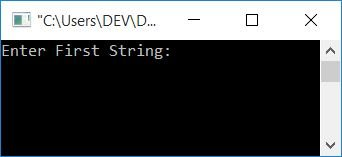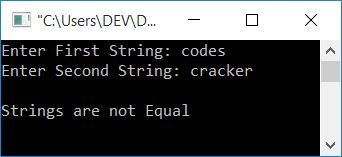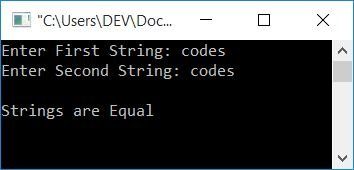# C Program to Compare Two Strings

In this article, you will learn and get code for checking whether the two input strings are equal or not, both with and without using a standard library function.

## Compare strings in C without using the strcmp() function

This program will not use any standard library function, such as strcmp(), that helps while comparing two strings in C. Rather, this program will compare the two given strings with the help of a self-defined code. Let's have a look at it:

```#include<stdio.h>
#include<conio.h>
int main()
{
char str1, str2;
int i=0, chk=0;
printf("Enter First String: ");
gets(str1);
printf("Enter Second String: ");
gets(str2);
while(str1[i]!='\0' || str2[i]!='\0')
{
if(str1[i]!=str2[i])
{
chk = 1;
break;
}
i++;
}
if(chk==0)
printf("\nStrings are Equal");
else
printf("\nStrings are not Equal");
getch();
return 0;
}```

This program was compiled and executed in the Code::Blocks IDE. On executing the above program, you will see the output as shown in the snapshot given below:Now supply any two strings, say "codes" and "cracker", and press the ENTER key to see the output as shown here in the snapshot given below:Let's take another sample run, in which let's suppose that the user has provided two equal strings, say codes and codes.Let's take a look at some of the main logic used in the above program.

#### Logic used in previous Program

Here is a list of some of the main logic used in the previous program:

• Using the gets() function, scan both the string from the user at run-time.
• The first and second strings should be saved in the str1 and str2 variables.
• Create a while loop that runs until the last character of both strings.
• Inside the loop, use an if statement to check whether the first character of the first string is not equal to the first character of the second string. If it evaluates to be true, then initialize 1 to chk and use the break keyword to terminate the loop.
• If the condition of the if block evaluates to be false, then the value of i gets incremented, and program flow goes back to the condition of the while loop.
• This will continue until the condition of the while loop evaluates to be false or the condition of the if block (inside the while loop) evaluates to be true.
• After terminating the loop, check whether chk holds 0 or not. If it holds, then statements of if block never run. So both strings are equal.
• Otherwise, if chk holds 1, then the statement of the if block is executed, so both strings are not equal.

For example, let's suppose that the user enters "codes" and "cracker" as two input strings. So the dry run of the above program using these two given strings as input is given below:

• The program declares two char variables, str1 and str2, each of which can hold up to 50 characters.
• The program declares another two variables, namely i and chk, with 0 as their initial value.
• The program will scan two strings as input using the gets() function.
• If the user enters any string that will be smaller than 50 characters, say "codes," then a null-terminated character (\0) automatically gets assigned after the last character (s) of the given string (codes). That is, if the user supplies codes as the first string, then
• str1 holds c
• str1 holds o
• str1 holds d
• str1 holds e
• str1 holds s
• str1 holds \0
• The program checks the condition of the while loop.
• At first run, while loop condition,
`str1[i]!='\0' || str2[i]!='\0'`
or
`str1!='\0' || str2!='\0'`
or
`'c'!='\0' || 'c'!='\0'`
is found to be true.Because in both cases, c is not equal to a null-terminated character (\0).
• So the program flow goes inside the while loop.
• The program examines the condition of the if block, that is,
`str1[i]!=str2[i]`
or
`c!=c`
evaluates to be false, so program flow does not go inside the if block.
• The program increments the value of i and goes back to the condition of the while loop again.
• The program continues running until the condition of the while loop evaluates to be false.
• Or inside the loop, if the condition of the if block evaluates to be true, then program flow goes inside the if block, and 1 gets initialized to the chk variable. Using the break keyword, the program flow exits the while loop or the loop is terminated.
• and checks whether chk holds its original value (0) or not. If it holds, then the if block's condition never evaluates to true, indicating that no character mismatch occurred from the same position of both strings.
• So the program prints "Strings are equal" as output. Otherwise, prints "Strings are not Equal."

## Using a Library Function to Compare Strings in C

Let's create another simple program that also checks whether the given two strings are equal or not using the standard library function C.

```#include<stdio.h>
#include<conio.h>
#include<string.h>
int main()
{
char str1, str2;
int len1, len2;
printf("Enter First String: ");
gets(str1);
printf("Enter Second String: ");
gets(str2);
len1 = strlen(str1);
len2 = strlen(str2);
if(len1==len2)
{
if(strcmp(str1, str2)==0)
printf("\nStrings are Equal");
else
printf("\nStrings are not Equal");
}
else
printf("\nStrings are not Equal");
getch();
return 0;
}```

This program will produce the same output as the previous program. In the above program, first we have checked whether the length of both strings is equal or not; if it is equal, then we process further. If the lengths of the two given strings are not equal, neither string will be equal.

Note: If both strings are equal, then the strcmp() function returns 0. Otherwise, if both strings are not equal, then the strcmp() function returns 1.

#### The same program in different languages

C Quiz

« Previous Program Next Program »